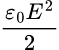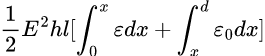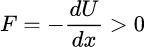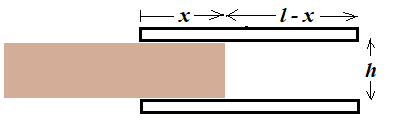# Why is a Dielectric slab ejected from the capacitor when energized?

• I
• theconfusedphysicist
In summary, a capacitor connected to a potential difference generator V consists of 2 square metal plates with a dielectric slab inserted between them. By calculating the derivative of the electrostatic energy with respect to the x-coordinate, it is determined that there is a force pulling the slab into the capacitor. However, when the experiment is conducted, it is found that there is actually a repulsive force pushing the slab out of the capacitor. This is due to the fact that the correct formula for the force is -dUtotal/dx, which takes into account the changes in energy in the DC generator. This results in the slab moving towards the right, as it is pulled into the space between the plates.f

#### theconfusedphysicist

TL;DR Summary
Dielectric slab in a capacitor connected at potential difference V.
Why is the slab thrown out?
A capacitor consisting of 2 square metal plates placed at a certain distance is connected to a potential difference generator V.

A slab of dielectric material is inserted into the space between the armatures.

By doing the calculation of the derivative of the electrostatic energy with respect to the x-coordinate, one obtains that the force acting on this slab is such as to pull it into the capacitor.

However, if you do the experiment, it turns out that the slab is thrown out of the capacitor by a repulsive force.

Why?

Thanks

TL;DR Summary: Dielectric slab in a capacitor connected at potential difference V.
Why is the slab thrown out?

By doing the calculation of the derivative of the electrostatic energy with respect to the x-coordinate, one obtains that the force acting on this slab is such as to pull it into the capacitor.
What is "the calculation" of "electrostatic energy". Please show explicitly how this derivative result is obtained, as some care is required.

•vanhees71 and TSny
However, if you do the experiment, it turns out that the slab is thrown out of the capacitor by a repulsive force.
I don't think this is correct. A partially inserted dielectric slab will always be pulled into a charged capacitor, not thrown out.

What is "the calculation" of "electrostatic energy". Please show explicitly how this derivative result is obtained, as some care is required.
The electric field between the armatures of the capacitor is: E=V/h (With h distance between capacitor armatures)

Next, the electrostatic energy is calculated as the integral ofin the volume.

So you get Energy U=so the force ish is the distance between the capacitor armatures
l is the side of the square armature
x is the position up to where the plate reaches

So you get Energy U= View attachment 327374
This expression for the electrostatic energy ##U_{\text{cap}}## stored in the capacitor is correct if ##d## and ##l## represent the same thing (the edge length of the square capacitor plates).

Evaluating the integrals in your expression, show that the capacitor energy can be written in terms of the potential ##V## as $$U_{\text{cap}}= \frac 1 2 C(x) V^2$$ where $$C(x) = \frac {l}{h} \left[(\varepsilon - \varepsilon_0)x + \varepsilon_0 \, l\right].$$ ##C(x)## is the capacitance of the capacitor when the slab is inserted a distance ##x##.Note that ##U_{\text{cap}}## increases when ##x## increases. That is, the energy in the capacitor increases if the movement of the slab is toward the right in the picture.

Since ##V## is held constant by the DC generator, $$\frac{dU_{\text{cap}}}{dx} = \frac 1 2 C'(x) V^2$$ where ##C'(x) = \large \frac{dC(x)}{dx}##.

Let ##F_x## be the electric force acting on the slab. If ##F_x > 0##, then the force on the slab is toward the right in the picture. If ##F_x < 0##, then the force is toward the left.

If ##F_x = -dU_{\text{cap}}/dx ##, then you can check that ##F_x =-\frac 1 2 C'(x) V^2 < 0## and the slab would be ejected.

However, it turns out that ##F_x \neq -U_{\text{cap}}/dx ##. Instead, there are energy changes that take place in the DC generator which must be taken into account. If ##x## increases by ##dx##, the generator must supply some charge ##dQ## to the capacitor in order to keep the potential of the capacitor fixed at ##V##. In doing this, the energy stored in the generator changes by ##dU_{\text{gen}} = - (dQ) V.##

The correct formula for ##F_x## is $$F_x = -dU_{\text{total}}/dx = -\left(dU_{\text{cap}}/dx+dU_{\text{gen}}/dx\right)$$
Use the capacitor formula ##Q = CV## to show $$dU_{\text{gen}}/dx = - (dQ/dx) V = -C'(x) V^2 .$$ Compare this with ##dU_{\text{cap}}/{dx}= \frac 1 2 C'(x) V^2 ##. Thus, if the slab moves toward the right, the generator loses energy at twice the rate that the capacitor gains energy. Overall, ##U_{\text{tot}}## decreases if the slab moves toward the right.

Finally, show that $$F_x =-U_{\text{total}}/dx = +\frac 1 2 C'(x) V^2.$$
Thus, ##F_x > 0##. Conclude that the slab does in fact move toward the right. The slab is pulled into the space between the plates.

•vanhees71 and theconfusedphysicist
This expression for the electrostatic energy ##U_{\text{cap}}## stored in the capacitor is correct if ##d## and ##l## represent the same thing (the edge length of the square capacitor plates).

Evaluating the integrals in your expression, show that the capacitor energy can be written in terms of the potential ##V## as $$U_{\text{cap}}= \frac 1 2 C(x) V^2$$ where $$C(x) = \frac {l}{h} \left[(\varepsilon - \varepsilon_0)x + \varepsilon_0 \, l\right].$$ ##C(x)## is the capacitance of the capacitor when the slab is inserted a distance ##x##.

View attachment 327417

Note that ##U_{\text{cap}}## increases when ##x## increases. That is, the energy in the capacitor increases if the movement of the slab is toward the right in the picture.

Since ##V## is held constant by the DC generator, $$\frac{dU_{\text{cap}}}{dx} = \frac 1 2 C'(x) V^2$$ where ##C'(x) = \large \frac{dC(x)}{dx}##.

Let ##F_x## be the electric force acting on the slab. If ##F_x > 0##, then the force on the slab is toward the right in the picture. If ##F_x < 0##, then the force is toward the left.

If ##F_x = -dU_{\text{cap}}/dx ##, then you can check that ##F_x =-\frac 1 2 C'(x) V^2 < 0## and the slab would be ejected.

However, it turns out that ##F_x \neq -U_{\text{cap}}/dx ##. Instead, there are energy changes that take place in the DC generator which must be taken into account. If ##x## increases by ##dx##, the generator must supply some charge ##dQ## to the capacitor in order to keep the potential of the capacitor fixed at ##V##. In doing this, the energy stored in the generator changes by ##dU_{\text{gen}} = - (dQ) V.##

The correct formula for ##F_x## is $$F_x = -dU_{\text{total}}/dx = -\left(dU_{\text{cap}}/dx+dU_{\text{gen}}/dx\right)$$
Use the capacitor formula ##Q = CV## to show $$dU_{\text{gen}}/dx = - (dQ/dx) V = -C'(x) V^2 .$$ Compare this with ##dU_{\text{cap}}/{dx}= \frac 1 2 C'(x) V^2 ##. Thus, if the slab moves toward the right, the generator loses energy at twice the rate that the capacitor gains energy. Overall, ##U_{\text{tot}}## decreases if the slab moves toward the right.

Finally, show that $$F_x =-U_{\text{total}}/dx = +\frac 1 2 C'(x) V^2.$$
Thus, ##F_x > 0##. Conclude that the slab does in fact move toward the right. The slab is pulled into the space between the plates.
Thanks, you are great :)

•berkeman
This expression for the electrostatic energy ##U_{\text{cap}}## stored in the capacitor is correct if ##d## and ##l## represent the same thing (the edge length of the square capacitor plates).

Evaluating the integrals in your expression, show that the capacitor energy can be written in terms of the potential ##V## as $$U_{\text{cap}}= \frac 1 2 C(x) V^2$$ where $$C(x) = \frac {l}{h} \left[(\varepsilon - \varepsilon_0)x + \varepsilon_0 \, l\right].$$ ##C(x)## is the capacitance of the capacitor when the slab is inserted a distance ##x##.

View attachment 327417

Note that ##U_{\text{cap}}## increases when ##x## increases. That is, the energy in the capacitor increases if the movement of the slab is toward the right in the picture.

Since ##V## is held constant by the DC generator, $$\frac{dU_{\text{cap}}}{dx} = \frac 1 2 C'(x) V^2$$ where ##C'(x) = \large \frac{dC(x)}{dx}##.

Let ##F_x## be the electric force acting on the slab. If ##F_x > 0##, then the force on the slab is toward the right in the picture. If ##F_x < 0##, then the force is toward the left.

If ##F_x = -dU_{\text{cap}}/dx ##, then you can check that ##F_x =-\frac 1 2 C'(x) V^2 < 0## and the slab would be ejected.

However, it turns out that ##F_x \neq -U_{\text{cap}}/dx ##. Instead, there are energy changes that take place in the DC generator which must be taken into account. If ##x## increases by ##dx##, the generator must supply some charge ##dQ## to the capacitor in order to keep the potential of the capacitor fixed at ##V##. In doing this, the energy stored in the generator changes by ##dU_{\text{gen}} = - (dQ) V.##

The correct formula for ##F_x## is $$F_x = -dU_{\text{total}}/dx = -\left(dU_{\text{cap}}/dx+dU_{\text{gen}}/dx\right)$$
Use the capacitor formula ##Q = CV## to show $$dU_{\text{gen}}/dx = - (dQ/dx) V = -C'(x) V^2 .$$ Compare this with ##dU_{\text{cap}}/{dx}= \frac 1 2 C'(x) V^2 ##. Thus, if the slab moves toward the right, the generator loses energy at twice the rate that the capacitor gains energy. Overall, ##U_{\text{tot}}## decreases if the slab moves toward the right.

Finally, show that $$F_x =-U_{\text{total}}/dx = +\frac 1 2 C'(x) V^2.$$
Thus, ##F_x > 0##. Conclude that the slab does in fact move toward the right. The slab is pulled into the space between the plates.
Not that this assumes that the voltage, ##V##, is constant, i.e., that the capacitor is connected to a "voltage source". You can solve for the same problem, when the charged capacitor is isolated, i.e., for the charge ##Q## on one of its plates (and ##-Q## on the other) being constant!

•TSny
Not that this assumes that the voltage, ##V##, is constant, i.e., that the capacitor is connected to a "voltage source". You can solve for the same problem, when the charged capacitor is isolated, i.e., for the charge ##Q## on one of its plates (and ##-Q## on the other) being constant!
Yes. The formula ##F_x = \frac 1 2 C'(x) V^2## is applicable to all cases. If the charge Q is kept constant then the force as a function of ##x## would be $$F_x = \frac 1 2 C'(x) V(x)^2 = \frac 1 2 \frac{C'(x)}{C(x)^2} Q^2$$ .

[EDIT: The following is wrong in view of #5 and #10]
The energy stays the same, i.e.,
$$E=\frac{1}{2} C(x) V^2=\frac{1}{2 C(x)} Q^2.$$
For the force you have for constant ##V##
$$F_x=-\partial_x E=-\frac{1}{2} C'(x) V^2$$
and for constant ##Q##
$$F_x=+\frac{1}{2} \frac{C'(x)}{C^2(x)} Q^2=+\frac{1}{2} C'(x) V^2(x).$$
Note that the direction of the force is the opposite in both cases, but also the magnitude is different since for constant ##Q## the voltage ##V(x)=Q/C(x)## depends on ##x##.

Last edited:
The energy stays the same, i.e.,
$$E=\frac{1}{2} C(x) V^2=\frac{1}{2 C(x)} Q^2.$$
For the force you have for constant ##V##
$$F_x=-\partial_x E=-\frac{1}{2} C'(x) V^2$$
For constant ##V##, the force is not given by ##F_x=-\partial_x E_{\text{cap}}##. It's given by ##F_x=-\partial_x \left(E_{\text{cap}} +E_{\text{source}}\right)##, where ##E_{\text{source}}## is the energy of the source (such as chemical energy in a battery).

You can show that if the slab moves inward in the positive-x direction while ##V## is kept constant, then the total energy ##( E_{\text{cap}} +E_{\text{source}})## stored in the source and the capacitor decreases. The source loses more energy than the capacitor gains. See post #5. If the slab moves outward in the negative-x direction, the total energy of the source and the capacitor increases.

Suppose there is no friction between the slab and the capacitor plates and the slab is released from rest from the position shown in the figure of post #5. The electric force on the slab will accelerate the slab, increasing the kinetic energy of the slab.

If the slab were to move in the negative x direction while the source is connected, then there would be an overall increase in total energy of the isolated system. That is ##\Delta\left( E_{\text{cap}} +E_{\text{source}}\right) + \Delta (KE_{\text{slab}}) > 0##, which violates energy conservation. So, the force on the slab must be in the positive-x direction and the slab is pulled into the space between the plates. The gain in KE of the slab is balanced by the decrease in ##(E_{\text{cap}} +E_{\text{source}})##.

If the source is removed before releasing the slab so that ##Q## is fixed, then the force is still in the positive-x direction. The increase in KE of the slab is accounted for by the decrease in ##E_{\text{cap}} = \large \frac{Q^2}{2C(x)}##.

-----
When the slab is inserted a distance ##x##, let ##Q(x)## be the charge on the capacitor and ##V(x)## be the potential of the capacitor. The relation between ##Q(x)## and ##V(x)## is ##Q(x) = C(x)V(x)##, where ##C(x)## is given in post #5.

The electric force on the slab for a given ##x## can be written as $$F_x(x) = \frac 1 2 C'(x) V(x)^2 = \frac 1 2 \frac{C'(x)}{C(x)^2} Q(x)^2$$ These expressions for a given ##x## do not depend on whether ##V## or ##Q## (or neither) is being held constant as ##x## varies. In all cases, the electric force is in the direction of increasing ##x## (until the slab is fully inserted).

If ##V## is fixed, then the formula ##F_x(x) = \frac 1 2 C'(x) V^2## is a nice way to express the force. Since ##C'(x)## is constant, the force remains constant as ##x## varies.

If ##Q## is fixed, then the formula ##F_x(x) =\frac 1 2 \frac{C'(x)}{C(x)^2} Q^2## is nice. Now the force decreases in magnitude as ##x## increases.

•vanhees71
I stand corrected! You are right, one has to take into account the changing charge on the plates for ##V=\text{const}## in the energy balance to calculate the force.